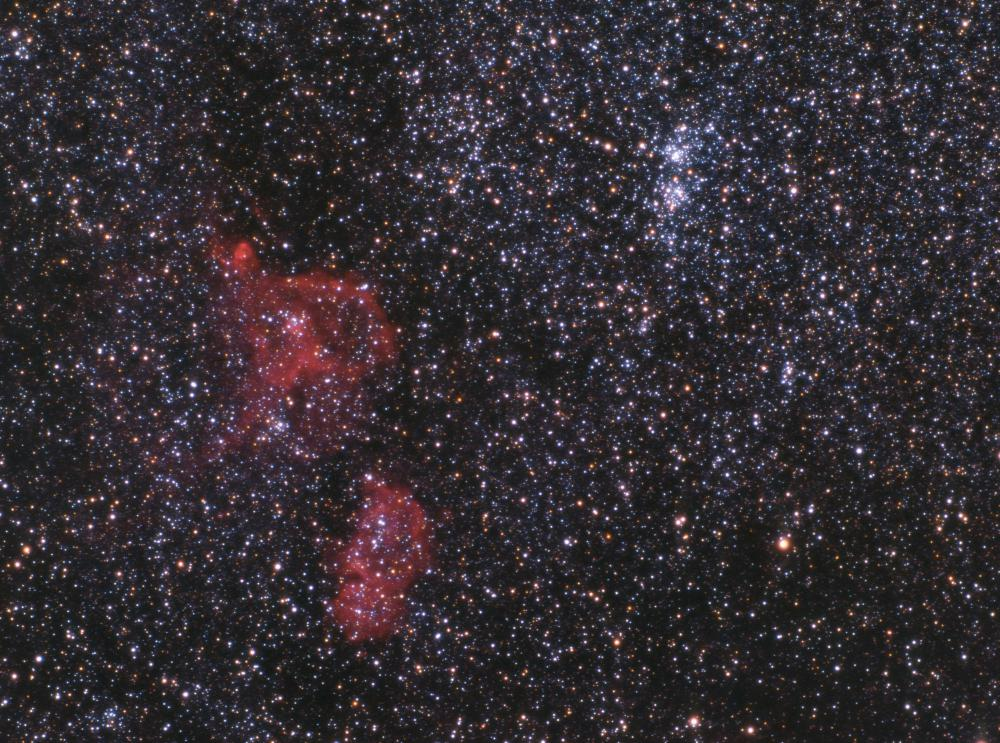# What Is the Initial Mass Function?

Ray Hawk
Ray HawkAccording to most astronomy estimates as of 2011, there exists somewhere between 200 billion and 400 billion stars in the Milky Way galaxy.

The initial mass function (IMF) was first derived in 1955 by Edwin Saltpeter, an Austrian astrophysicist, and is a method of calculating the range of different masses for stars that will form out of condensing gasses in space. It is a form of probability distribution that uses complex mathematics and physics equations with a base value of one solar mass, which represents the mass of Earth's Sun as a stepping off point for the range of other stars that will form. The premise of the initial mass function in stellar astronomy is that it is much more common and likely for stars of low mass to form in space than it is for stars of high mass, with stars that have approximately 0.5 solar masses being the most common in the Milky Way galaxy as of 2011. Despite this fact, the rarest of stars, at about 60 solar masses in size or higher, contribute most of the visible light to the Milky Way galaxy.

According to most astronomy estimates as of 2011, there exists somewhere between 200,000,000,000 and 400,000,000,000 stars in the Milky Way galaxy. The initial mass function predicts that the probability for the majority of these stars is that they are 0.9 solar masses or less, while less than 1% of them make up sizes ranging from 8 to 120 solar masses. The IMF calculates masses based on when each star first formed, and most stars begin as dwarf stars of only 0.085 to 0.8 solar masses. As these main sequence stars age, they tend to lose mass and gain volume.

Despite widely varying conditions in substellar regions of space where stars form, the power laws of the initial mass function have proven to be true. This means that, whether star formation is occurring in small molecular clouds of gas or in dense star clusters, the same distribution of star ranges arises regardless. These observations conflict with star formation theories as of 2011 due to conditions such as the fact that, in a metal dense region of space, the star distribution should include a greater percentage of massively large stars.

It is estimated that, in about 5,000,000,000 years, the Sun itself will expand as it burns away its hydrogen fuel and begins to fuse helium to heavier elements. At this stage, the Sun will fill a volume of space reaching to the orbit of the Earth for about 20% of its total life span, and retain 50% of its previous mass as a red giant. As small stars like the Sun age and lose mass in the process, they increasingly bias the initial mass function more towards the small mass end of the spectrum, in large part because there are far more small stars in existence.

## You might also Like